# Subtraction With Regrouping

Solve more complex subtraction problems with regrouping, also known as borrowing or carrying!Author
Taylor HartleyExpert Reviewer

Published: August 24, 2023# Subtraction With Regrouping

Solve more complex subtraction problems with regrouping, also known as borrowing or carrying!Author
Taylor HartleyExpert Reviewer

Published: August 24, 2023# Subtraction With Regrouping

Solve more complex subtraction problems with regrouping, also known as borrowing or carrying!Author
Taylor HartleyExpert Reviewer

Published: August 24, 2023Key takeaways

• Subtraction with regrouping is a key building block for learning mathematics – It allows you to solve double and triple digit subtraction problems.
• It’s important to learn subtraction with regrouping – That way, you can better understand and solve complex word problems.
• Subtraction with regrouping is also referred to as borrowing or carrying –  It’s a skill you’ll need for higher level math.

Subtraction is one of the most important building blocks for learning more complicated math problems. Subtracting 8 from 10 gives us 2, which is pretty easy to follow, but what happens when we need to solve multi-digit subtraction problems?

To prepare for more involved subtraction problems, we can use a handy skill called regrouping. Subtraction with regrouping lets you tackle problems where the number you must subtract from is smaller than the number you are subtracting.

We know that sounds confusing right now, but once we work through a few examples together, you’ll get the hang of it in no time.

## What is subtraction with regrouping?Subtraction with regrouping, also called borrowing or carrying, is used to solve subtraction problems where the equation is written vertically This might look something like: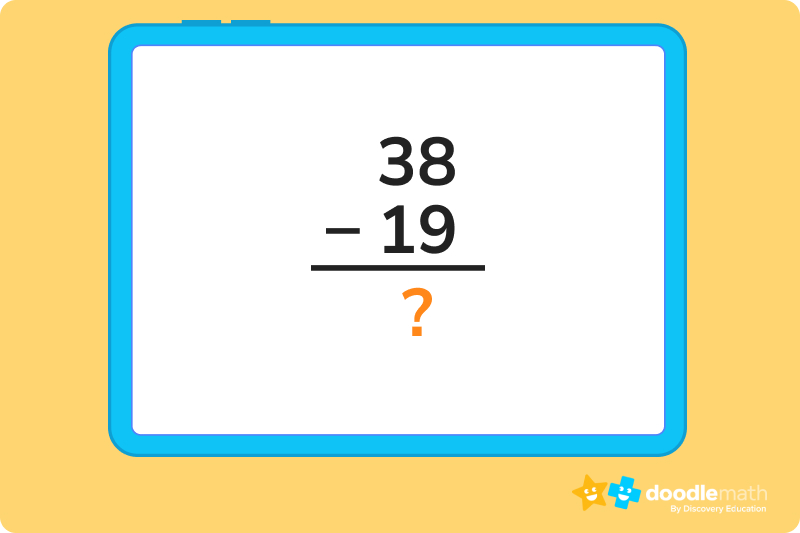See how, in the top number, the 8 in the 1s place is smaller than the 9 in the 1s place in the bottom number? This means we can’t subtract…unless we use regrouping!

Let’s look at some terms that will help us better understand this skill.

## Important terms to knowThese terms are going to be an important part of understanding regrouping, so pay close attention. We’ll be discussing three different terms: subtrahends, minuends, and differences.

• Subtrahend – The number we subtract from the minuend.
• Minuend – The number we’re subtracting another number from.
• Difference – The end value of subtracting the subtrahend from the minuend.

These terms will appear in the following format every time you subtract:

Minuend – Subtrahend = Difference

For example, let’s take a look at this subtraction equation: 14 – 5 = 9

In this instance, the minuend would be 14, the subtrahend would be 5, and the difference would be 9.

## How to subtract with regrouping, in 5 easy stepsNow, let’s walk through the basic steps of how to use regrouping when we are subtracting. This involves setting up our subtraction equation using the column method.

After we do that, we will identify which numbers are in which place values, then finish with our borrowing/carrying. Once we’ve borrowed from the tens place and carried to the ones, it’ll make it much easier to subtract.

Let’s take a look at the following problem:

23 – 8 = 15

### 1. Place the numbers you are subtracting verticallyFirst, we need to set up our equation in vertical form. This will make it easier for us to borrow our numbers and show our work in the process. Just write the 8 write under your 23, but make sure the 8 falls right under the 3 within the ones place.

It should look like this: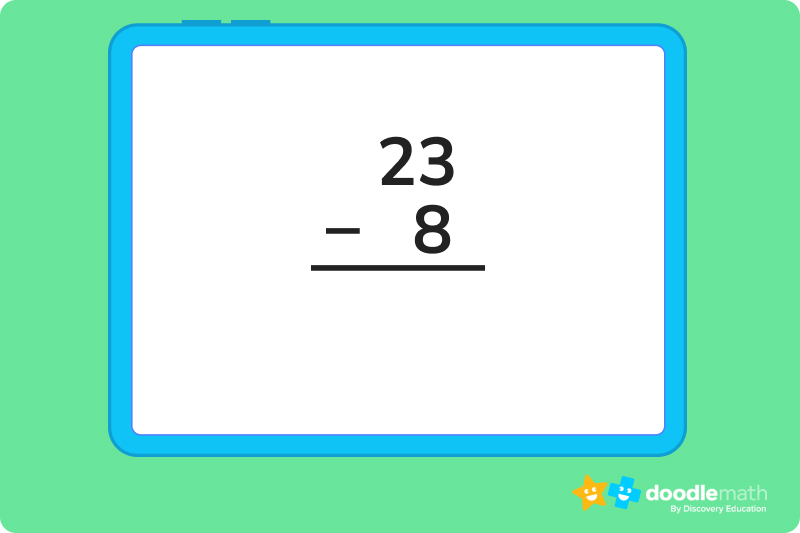### 2. Read the problem and look at the different place valuesNow, let’s identify which numbers are in which place values. This will be important for knowing which number to borrow from within the minuend, and where it should be carried.

You should always be borrowing from the tens place, and carrying to the ones. For our minuend 23, 2 is in the tens place and 3 is in the ones.

### 3. Since we can’t subtract 8 from 3, borrow 1 from the tens columnHere is the most important step of this process. Now, we just have to borrow one from the tens column and carry it into the ones. We will always only borrow 1 from the tens column, remember that!

So the equation becomes: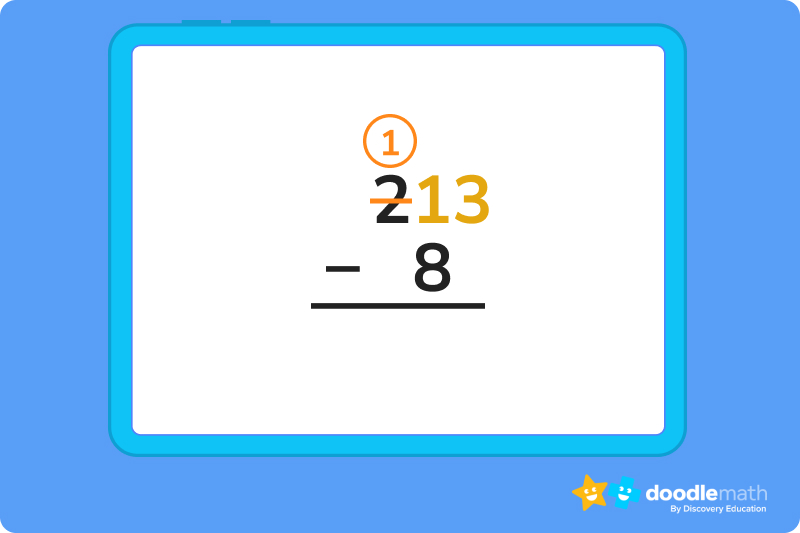### 4. Now, do your subtraction, first on the ones sideNow that we’ve taken 1 away from the tens column, we only have 1 left in the tens column. However, we can write that same 1 right next to the three in the ones column, making 13.

### 5. Then, subtract from the tens sideNow, we’re ready to do our subtraction. Since we turned the three in the ones column into a thirteen in the step before, we can subtract 8 from 13 instead of 8 from 3!

13 minus 8 equals 5, and the 1 left over in the tens column has no subtrahend, so it stays the same. That means we have a 1 in the tens column and a 5 in the ones column, giving us a difference of 15.

## Let’s practice together!Here’s three practice problems we can work through together to make sure you have the basics down.

1. Write 24 – 5 as a vertical equation

a. We know that the minuend is 24, and the subtrahend is 5, which means 24 goes on the top of the equation with 5 on the bottom.

b. We also know that both 4 and 5 are in the ones place, meaning they should be placed in the same column.

c. Now, we’re ready to write our finished equation:

24

-5

2. Simon got 35 toy cars for his birthday this year. His brother Charles also loves toy cars, so Simon decides to give Charles 6 cars as a present. How many cars is Simon left with?

a. Let’s start by identifying our minuend and subtrahend. The number we’re subtracting from is 35, and the number being subtracted is 6.

b. That means we’ll need to set up our column method with 35 on the top, and 6 right below it.

c. We also know that both 5 and 6 are in the ones place, meaning they should be put in the same column. The setup for our vertical equation should look like this:

35

-6

d. Now that we have our equation, we can do our regrouping. First, we’ll borrow the one from the tens column of the 35, and carry it into the ones column.

e. That means we subtract 1 from the 3, leaving a two in the tens column. We write that one next to the 5 in the ones column, giving us a 15.

f. Now we can subtract 6 from 15 much easier, giving us a difference of 9 in the ones column. And since we aren’t subtracting anything from the 2, we can place that 2 in the tens column, giving us a total difference of 29.

3. Robert has 21 marbles in his box. His sister Margaret decides to take 3 marbles for herself. How many marbles is Robert left with?

a. Here, we’ll need to set up our vertical equation with 21 on the top, and 3 on the bottom. 21 is the minuend, here, and 3 is the subtrahend. It should look like the following:

21

-3

b. Since we’ve lined up the 3 and the 1 in the ones place, we’re ready to regroup. We’ll borrow a 1 from the tens place, and carry it into the ones, leaving a one leftover in the tens place, and an 11 in the ones.

c. Now we can subtract 3 from 11 in the ones column, giving us 8, and drag the 1 in the tens place down to form a difference of 18.

Ready to give it a go?

Now it’s time for you to give a few of these problems a shot on your own. Feel free to consult the explanations, definitions, and practice problems above if you get confused or stumped on a question. And remember, practice makes perfect, so don’t get discouraged. Keep working through these problems and we promise you’ll get the hang of regrouping in no time!

## Parent Guide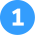13

-7

How did we get here?

1. We’ve identified that the minuend is 13, while the subtrahend is 7.
2. That means we can set up our vertical equation with the minuend (13) on top, and the subtrahend (7) on the bottom.
3. We also know that both 3 and 7 are in the ones place, so they should be in the same column within our equation. This gives us the vertical equation written above.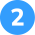8

How did we get here?

1. First, we need to identify that the 3 and the 5 are in the ones place, so we’ll be subtracting 5 from 3 in this equation.
2. 5 is greater than 3, so we know we’ll need to borrow a one from the tens column so we can actually subtract.
3. So, we borrow a 1 from the tens column and place it next to the 3 in the ones column, making thirteen. Since we’ve technically subtracted 1 from the tens column, the two there becomes a one.
4. Now, we can subtract in the ones column. 13 – 5 = 8.
5. The tens column is left as 1 – 1, which gives us a difference of 0. That means the total difference for this problem is 8.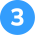45

-17

How did we get here?

1. First, we identify the minuend and the subtrahend so that we know which number to place on top, and which number to put on the bottom.
2. Since we’re subtracting 17 cups away from the 45 already in the cabinet, we know that the minuend is 45 and the subtrahend is 17.
3. We also need to identify which numbers are in which places. Both 4 and 1 are in the tens place, and 5 and 7 are in the ones, so these numbers should be in the same respective columns.
4. We’re now ready to write our formula.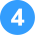18

How did we get here?

1. Here, we know that the minuend is 32 and the subtrahend is 14, since we are taking away 14 pencils from the total of 32.
2. That means we can set up the following vertical equation:
32
-14
3. Since the 4 in the ones column is bigger than the 2, we know we’ll need to regroup. Now, we’ll borrow a one from the tens column and carry it to the ones, giving us a 12 to subtract the four from.
4. The 3 in the ones column loses the 1 we borrowed, decreasing it to 2.
5. Now, we can subtract the 1 from the 2 in the tens column, and the 4 from the 12 in the ones column.
6. 2 – 1 = 1, and 12 – 4 = 8. We now write the 1 in the tens and the 8 in the ones, giving us a difference of 18.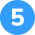14

How did we get here?

1. The minuend for this problem is 22, and the subtrahend is 8. That means the 22 goes on top, and the 8 goes underneath it for our vertical equation.
2. Since the 2 and the 8 are both in the ones column, we know we’ll have to borrow from the tens column, since 8 is greater than 2.
3. That means we borrow a one, leaving a 1 leftover in the tens column, and carry it to the ones column, giving us a 12.
4. Now we can solve 12 – 8, giving us 4. We aren’t subtracting anything from the 1 in the minuend since the subtrahend has no value in the tens column. We can simply write the 1 next to our 4 for a solved difference of 14.

## FAQs about math strategies for kidsWe understand that diving into new information can sometimes be overwhelming, and questions often arise. That’s why we’ve meticulously crafted these FAQs, based on real questions from students and parents. We’ve got you covered!

If you know how to do subtraction with regrouping – also known as borrowing or carrying – then you will be able to subtract any number from any other number.

This will help you as you progress from more basic level mathematics to more complicated mathematics!

Look at the number in the 10s place as being the number of 10s that exist in the number itself.

So, let’s say we have the number 24. To make the number 20, you need two 10s, right? That’s why there is a 2 in the 10s place!

Negative numbers do exist! When you’re subtracting a large number from a smaller number, you will get a negative answer. For example, 13 – 17 = -4, right?

But, when we use subtraction with regrouping, we are almost always subtracting a smaller number from a larger number. For example, in 13 – 4, 13 is still larger than 4. The issue is that, when you stack these numbers vertically, 3 is smaller than 4, so we need to borrow to make it easier to calculate.

When we borrow from the 10s column, the 3 becomes 13, and 13 – 4 is 9.## Related Posts

Title 1

Title 2

Title 3

Lesson credits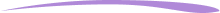Taylor Hartley

Taylor Hartley is an author and an English teacher based in Charlotte, North Carolina. When she's not writing, you can find her on the rowing machine or lost in a good novel.

Jill Padfield has 7 years of experience teaching high school mathematics, ranging from Alegra 1 to AP Calculas. She is currently working as a Business Analyst, working to improve services for Veterans while earning a masters degree in business administration.

Taylor Hartley

Taylor Hartley is an author and an English teacher based in Charlotte, North Carolina. When she's not writing, you can find her on the rowing machine or lost in a good novel.

Jill Padfield has 7 years of experience teaching high school mathematics, ranging from Alegra 1 to AP Calculas. She is currently working as a Business Analyst, working to improve services for Veterans while earning a masters degree in business administration.# Are you a parent, teacher or student?

Are you a parent or teacher?

## Hi there!

Book a chat with our team